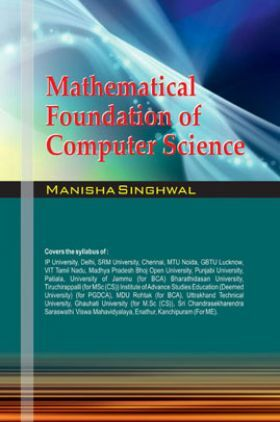# Mathematical Foundation of Computer Science

Sold ( 54 times )
11519 Views
MRP : ₹295.00
Price : ₹280.25
You will save : ₹14.75 after 5% Discount
Inclusive of all taxes

 Buy Ebook₹280.25 Rental Ebook Up to 72% OffFlat 72% Off

Save extra with 2 Offers

Get ₹ 50

Instant Cashback on the purchase of ₹ 400 or above

Product Specifications

 Publisher ASIAN All Computer Science books by ASIAN ISBN 9788184121896 Author: Manisha Singhwal Number of Pages 392 Available Available in all digital devicesMathematical Foundation of Computer Science

Mathematical Foundations of Computer Science explains the Fundamental Concepts in Mathematics. It can be used by the students in Computer Science as an introduction to the underlying ideas of mathematics for computer science. This book is designed to provide an introduction to some Fundamental Concepts in Discrete Mathematics and Automata. Mathematical Foundation of Computer Science is a compulsory paper in most computing program universities of our country. It covers Set Theory, Relation, Function Mathematical Induction, and Recurrence Relation generating Function, Algebraic structure, Lattices, Graph Theory, Finite Automation, Regular Expression, Turing Machine, and Formal Language. Each topic in the book has name treated as easy manner. A set of exercise (with answers) has also been given at the end of each chapter to test the student’s comprehension. The proofs of various Theorems and Examples have been given with enough details. Numerous Solved Examples have been included in the book to help the students in understanding various concepts easily. I have tried my best to keep the book free from misprints. I will be grateful to the readers who point out errors and omissions which in spite of all case, might have been there.

Contents:

1. Set Theory

2. Relation

3. Function

4. Mathematical Induction

5. Algebraic Structure

6. Ring and Fields

7. Propositional Logic

8. Boolean Algebra

9. Posets, Hasse Diagram and Lattices

10. Graph Theory

11. Theory of Computation

12. The Theory of Automata

13. Regular Expression

14. Formal Languages

15. Context Free Grammar

16. Pushdown Automata

17. Turing Machine